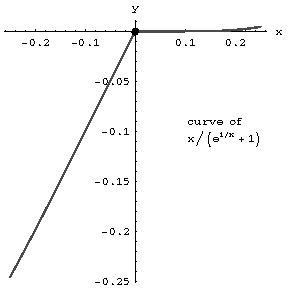# Breaking point

A singular point of a plane curve with the property that two branches of the curve end in it in such a way that each has a (one-sided) tangent at the point different from the other. For example, the origin is a breaking point of the curve $y=x/(1+e^{1/x})$ (see Fig.). The left and right derivatives are different at a breaking point.